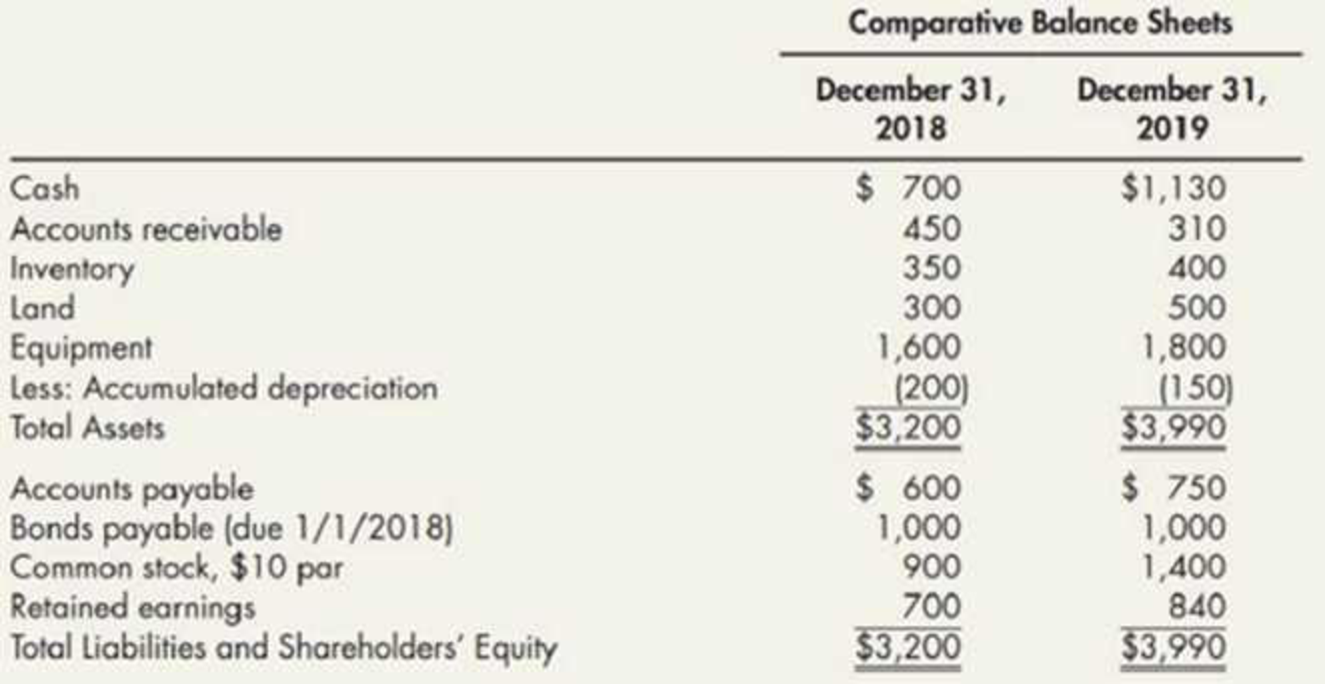Chapter 21, Problem 18E### Intermediate Accounting: Reporting...

3rd Edition
James M. Wahlen + 2 others
ISBN: 9781337788281

#### Solutions

Chapter
Section### Intermediate Accounting: Reporting...

3rd Edition
James M. Wahlen + 2 others
ISBN: 9781337788281
Textbook Problem
89 views

# Spreadsheet and Statement The following 2019 information is available for Stewart Company:Partial additional information: The equipment that was sold for cash had cost $400 and had a book value of$300. Land that was sold brought a cash price of $530. Fifty shares of stock were issued at par.(Appendix 21.1) Operating Cash Flows Spreadsheet Method Refer to the information for Stewart Company in E21-11.Required:Raised only on the information presented and using the direct method, prepare the cash flows from operating activities section of the 2019 statement of cash flows for Stewart using the spreadsheet method. To determine Prepare operating activities section in the cash flow statement under direct method of S Company for the year 2019. Explanation Prepare operating activities section in the cash flow statement under direct method: Table (1) Table (2) Working Note: (a) Net income for the year ended 2019 is$1,140.

(b) Calculate the decrease in accounts receivable.

Decrease in accounts receivable = (opening accounts receivable – closing accounts receivable) = $450-$310=$140 (c) Calculate an increase in inventory. Increase in inventory = Closing inventory - opening inventory=$450-$310=$50

(d) Calculate the cost of land sold.

Cost of land sold = (Proceeds from sale of land - Gain on sale of land)=$530$400=$130 (e) Calculate the purchase of land. Step 1: Calculate the balance amount of land after sale. Balance amount of land after sales)={Opening balanceSale of land}=$300$130=$170

Step 2: Calculate the purchase of land.

Purchase of land = (Closing balanceBalance amount of land after sale)=$500$170=$330 (f) Calculate the Proceeds from Equipment. Proceeds from sale of equipment = Book value Selling cost=$300$260=$40

(g) Calculate the Purchase of equipment.

Step 1: Calculate the balance amount of equipment after sales.

Balance amount of equipment after sales)={Opening balanceSale of equipment}=$1,600$400=$1,200 Step 2: Calculate the purchase of equipment. Purchase of Equipment = (Closing balanceBalance amount of equipment after sale)=$1,800$1,200=$600

(h) Calculate the Depreciation expense.

Depreciation Expenses = (Opening accumulated depreciation Closing accumulated depreciation)=$200$150=$50 (i) Calculate an increase in accounts payable. Increase in accounts payable = (Closing balance of accounts payableOpening balance of accounts payable)=$750$600=$150

(j) Calculate the proceeds from issuance of common stock.

Proceeds from issuance of common stock = Number of share×Value of share=50×$10=$500

(k) Calculate the payment of dividend

### Still sussing out bartleby?

Check out a sample textbook solution.

See a sample solution

#### The Solution to Your Study Problems

Bartleby provides explanations to thousands of textbook problems written by our experts, many with advanced degrees!

Get Started

#### Should firms require higher rates of return on foreign projects than on identical projects located at home? Exp...

Fundamentals of Financial Management, Concise Edition (with Thomson ONE - Business School Edition, 1 term (6 months) Printed Access Card) (MindTap Course List)

#### Define internal control.

Survey of Accounting (Accounting I)

#### What are transferred-in costs?

Cornerstones of Cost Management (Cornerstones Series)